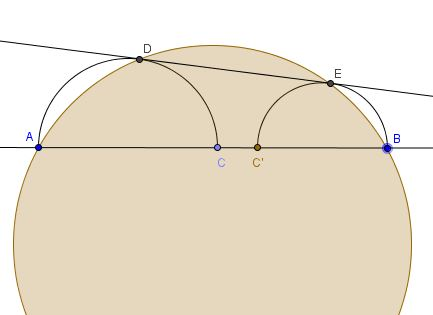# Concyclicity in Two Semicircles

### Problem

Point $C$ is taken on segment $AB;$ form two semicircles with centers $F$ and $G$ on $AC$ and, respectively, $BC$ as diameters. Let $DE$ be their common tangent.Prove that $A,$ $D,$ $E,$ and $B$ are concyclic.

### Hint

This configuration has already been considered elsewhere. The right triangles $ADC,$ $CEB,$ $DCE$ are similar which lays a way of computing the angles $ADE$ and $DEB.$ These can be used to prove that the quadrilateral $ABED$ is cyclic.

### Solution

Connect $AD,$ $CD,$ $CE,$ $BE.$ Observe that inscribed $\angle CAD$ equals $\angle CDE$ formed in circle $(F)$ by chord $DC$ and the tangent at $D.$ Similarly, $\angle CBE=\angle CED.$So there are three similar right triangles $ADC,$ $CEB,$DCE.$Further,$\begin{align} \angle ADE + \angle ABE &= (90^{\circ}+\angle CDE) + \angle ABE \\ &= 90^{\circ}+(\angle BCE + \angle ABE) \\ &= 90^{\circ}+90^{\circ}=180^{\circ}, \end{align}$implying that the quadrilateral$ABED$is indeed cyclic. In passing, note that the center$J$of$(ABED)$lies on the intersection of the perpendicular bisectors of$AB,AD,$and$BE$(as these are also chords in$(ABED)$) and the semicircle on$FG$(because$\Delta FGJ\$ is right (and similar to the above mentioned right triangles.)

### Acknowledgment

The problem is an auxiliary lemma from a bigger work by Emmanuel Antonio José García. Antonio also observed that the proof never uses the fact that the semicircles are touching. Hence, two additional cases: